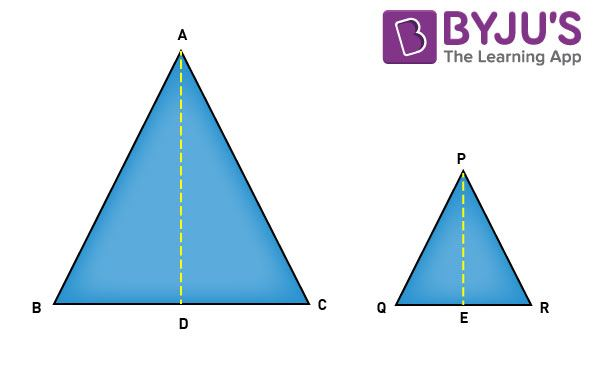Checkout JEE MAINS 2022 Question Paper Analysis : Checkout JEE MAINS 2022 Question Paper Analysis :

# Area Of Similar Triangles

Before learning the area of a similar triangle, let us first recall the similarity conditions. The two triangles are similar  to each other if,

• Corresponding angles of the triangles are equal
• Corresponding sides of  the triangles are in  proportion

If there are two triangles say ΔABC and ΔPQR, then they are similar if,

i)    ∠A=∠P, ∠B=∠Q and ∠C=∠R

ii)

$$\begin{array}{l} \frac {AB}{PQ} \end{array}$$
=
$$\begin{array}{l} \frac {BC}{QR} \end{array}$$
=
$$\begin{array}{l} \frac {AC}{PR} \end{array}$$

If we have two similar triangles, then not only their angles and sides share a relationship but also the ratio of their perimeter, altitudes, angle bisectors, areas and other aspects are in ratio.

In the upcoming discussion, the relation between the area of two similar triangles is discussed.

## Area of Similar Triangles Theorem

Theorem: If two triangles are similar, then the ratio of the area of both triangles is proportional to the square of the ratio of their corresponding sides.

To prove this theorem, consider two similar triangles ΔABC and ΔPQR;

According to the stated theorem,

$$\begin{array}{l}\large \frac {area ~of~ ΔABC}{area~ of~ ΔPQR}\end{array}$$
=
$$\begin{array}{l}\large \left(\frac{AB}{PQ}\right)^2 \end{array}$$
=
$$\begin{array}{l}\large \left(\frac{BC}{QR}\right)^2 \end{array}$$
=
$$\begin{array}{l} \large \left(\frac{CA}{RP}\right)^2 \end{array}$$

As, Area of triangle =

$$\begin{array}{l} \frac 12 \end{array}$$
× Base × Height

To find the area of ΔABC and ΔPQR, draw the altitudes AD and PE from the vertex A and P of ΔABC andΔPQR, respectively, as shown in the figure given below:Now, area of ΔABC =

$$\begin{array}{l} \frac 12 \end{array}$$

area of ΔPQR =

$$\begin{array}{l} \frac 12 \end{array}$$
× QR × PE

The ratio of the areas of both the triangles can now be given as:

$$\begin{array}{l}\large \frac{area~ of~ ΔABC}{area~ of~ ΔPQR} \end{array}$$
=
$$\begin{array}{l} \large \frac{ \frac 12 × BC × AD}{ \frac 12 × QR × PE}\end{array}$$

$$\begin{array}{l} \large \frac{area~ of~ ΔABC}{area ~of~ ΔPQR} \end{array}$$
=
$$\begin{array}{l} \large \frac{BC~×~AD}{QR~×~PE} \end{array}$$
……………. (1)

Now in ∆ABD and ∆PQE, it can be seen that:

∠ABC = ∠PQR (Since ΔABC ~ ΔPQR)

∠ADB = ∠PEQ (Since both the angles are 90°)

From AA criterion of similarity ∆ADB ~ ∆PEQ

$$\begin{array}{l} \frac {AD}{PE}\end{array}$$
=
$$\begin{array}{l} \frac {AB}{PQ}\end{array}$$
…………….(2)

Since it is known that ΔABC~ ΔPQR,

$$\begin{array}{l} \frac {AB}{PQ}\end{array}$$
=
$$\begin{array}{l} \frac {BC}{QR}\end{array}$$
=
$$\begin{array}{l} \frac {AC}{PR}\end{array}$$
…………….(3)

Substituting this value in equation (1), we get

$$\begin{array}{l} \large \frac{area~ of~ ΔABC}{area ~of~ ΔPQR} \end{array}$$
=
$$\begin{array}{l} \large \frac {AB}{PQ}\end{array}$$
×
$$\begin{array}{l} \frac {AD}{PE}\end{array}$$

Using equation (2), we can write

$$\begin{array}{l} \large \frac{area~ of~ ΔABC}{area ~of~ ΔPQR} \end{array}$$
=
$$\begin{array}{l} \large \frac {AB}{PQ}\end{array}$$
×
$$\begin{array}{l} \frac {AB}{PQ}\end{array}$$

$$\begin{array}{l} \large \frac{area~ of~ ΔABC}{area ~of~ ΔPQR} \end{array}$$
=
$$\begin{array}{l} \large \left( \frac {AB}{PQ}\right)^2\end{array}$$

Also from equation (3),

$$\begin{array}{l} \large \frac {area ~of~ ΔABC}{area~ of~ ΔPQR}\end{array}$$
=
$$\begin{array}{l} \large \left(\frac{AB}{PQ}\right)^2 \end{array}$$
=
$$\begin{array}{l} \large \left(\frac{BC}{QR}\right)^2 \end{array}$$
=
$$\begin{array}{l} \large \left(\frac{CA}{RP}\right)^2 \end{array}$$

This proves that the ratio of the area of two similar triangles is proportional to the squares of the corresponding sides of both the triangles.

#### To Know how to Find the Area Of Similar Triangles, Watch The Below Video:To have a better insight consider the following example.

### Example

Example 1: In ΔABC andΔAPQ, the length of the sides are given as AP = 5 cm , PB = 10cm and BC = 20 cm. Find the ratio of the areas of ΔABC and ΔAPQ.Solution:  In ΔABC and ΔAPQ , ∠PAQ is common and ∠APQ = ∠BAC (corresponding angles)

⇒ ΔABC ~ ΔAPQ  (AA criterion for similar triangles)

Since both the triangles are similar, using the theorem for areas of similar triangles we have,

$$\begin{array}{l} \large \frac {area ~of~ ΔABC}{area~ of~ ΔAPQ}\end{array}$$
=
$$\begin{array}{l} \large \left( \frac {AB}{AP} \right)^2 \end{array}$$
$$\begin{array}{l}\large \left( \frac {15}{5} \right)^2 \end{array}$$
= 9